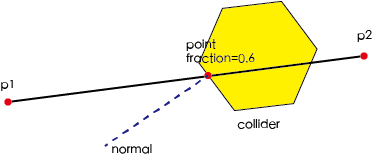# Physics Manager

The physics system takes Box2d as an internal physics engine and hides most of the Box2d implementation details (such as creating rigidbody, synchronizing rigidbody information to nodes). You can access some of the commonly used functions of Box2d through physics manager, such as click test, ray test, set test information, and so on.

### Enable Physics manager

The physics manager is disabled by default. If you need to use the physics system related functions, the first thing you need to do is enable the physics manager, otherwise all the physics object you setup in the editor will not produce any effect.

``````cc.director.getPhysicsManager().enabled = true;
``````

### Draw physics debugging information

The physics system does not draw any debugging information by default. If you need to draw debugging information, use `debugDrawFlags`. The physics system provides a variety of debugging information, you can combine the information to draw the relevant content.

``````cc.director.getPhysicsManager().debugDrawFlags = cc.PhysicsManager.DrawBits.e_aabbBit |
cc.PhysicsManager.DrawBits.e_pairBit |
cc.PhysicsManager.DrawBits.e_centerOfMassBit |
cc.PhysicsManager.DrawBits.e_jointBit |
cc.PhysicsManager.DrawBits.e_shapeBit
;
``````

Set the drawing flag to `0` to disable drawing.

``````cc.director.getPhysicsManager().debugDrawFlags = 0;
``````

### Conversion physics units to pixel units

Box2d uses meter - kilogram - second (MDS) unit system, it has the best performance operating under such a unit system. But we use pixel unit of length in 2D games, so we need a ratio to convert the physics units to the pixel units. In general, we set this ratio to `32`, which can be obtained by `cc.PhysicsManager.PTM_RATIO`, and this value is read-only. Usually the user does not need to care about this value, the physics system will automatically convert the physics units and pixel units to each other. User can use the familiar pixel units for all the calculations.

### Set physics gravity

Gravity is a very important thing in physics operation, and most physics games use the gravity as a important feature. The default gravity is (0, -320) pixels per second^2, according to the conversion rules described above, that's (0, -10) m / s^2 in physics unit.

If you want the gravity to be 0, you can set like this:

``````cc.director.getPhysicsManager().gravity = cc.v2 ();
``````

If you want to modify the gravity to other values, such as 640 pixels / second^2, then you can set like this:

``````cc.director.getPhysicsManager().gravity = cc.v2 (0, -640);
``````

## Query physics object

Often you may want to know which physics objects are in a given scene. For example, if a bomb explodes, objects in the range will be damaged; or in a strategy game, you may want to let the user drag to move a unit from a certain range.

The physics system provides several ways to efficiently and quickly look for objects in a region, each of which uses different ways to query objects that fit the needs of the game.

### Point test

The point test will test if there's a collider contains a specific point under the world coordinate system. If the test is successful, it will return the collider. If there're multiple collider that contains the point, a random one will be returned.

``````var collider = cc.director.getPhysicsManager().testPoint(point);
``````

### Rectangle test

The rectangle test will test if there's a specified rectangle (in world coordinate system) that intersect with the bounding box of a collider. If successful the collider will be added to the return list.

``````var colliderList = cc.director.getPhysicsManager().testAABB(rect);
``````

### Ray test

Ray detection is used to detect which colliders a given line passes through. We can also obtain the normal vector at the point where the given line passes through and other useful information.

``````var results = cc.director.getPhysicsManager().rayCast (p1, p2, type);

for (var i = 0; i <results.length; i ++) {
var result = results [i];
var collider = result.collider;
var point = result.point;
var normal = result.normal;
var fraction = result.fraction;
}
``````

The last parameter of the `rayCast` function specifies the type of detection, and the ray detection supports four types. This is because the ray detection of Box2d is not detected from the nearest object of the ray starting point, so the result of the test can not guarantee that the result is sorted by the distance from the object near the start of the ray. Cocos Creator's physics system will determine whether the Box2d test results are sorted based on the type of detection. This type will affect the result return to user.

• `cc.RayCastType.Any`: will detect any collider on the ray path. Once it detects any collider, it will immediately end the detection process and will no longer detect other objects.

• `cc.RayCastType.Closest`: will detect the nearest collider on the ray path, which is the default for the `rayCast` detection, slightly slower than above method.

• `cc.RayCastType.All`: will detect all colliders on the ray path, the order of the detected results is not fixed. In this type of detection, a collider may return multiple results because Box2d run the detection by testing the fixture, and a collider may consist of multiple fixtures. This is a more costly method and will be slower than above methods. More details can be found in the Physics Collider Component documentation.

• `cc.RayCastType.AllClosest`: All colliders on the ray path are detected, but the return result is filtered and only the relevant information about the nearest point of each collider is returned, the slowest method of all.

#### The result of ray detection

The results of ray detection contain a lot of useful information, you can utilize these info according to the actual need.

• `collider`: Specifies which collider the ray passes through.

• `point`: Specifies the point at which the ray intersects the collider.

• `normal`: Specifies the normal vector of the surface of the collider at the intersection.

• `fraction`: Specifies the score of the intersection point at the ray.

You can have a better understanding of the result of ray detection with the following figure.